# 1478 and Level 1

### Today’s Puzzle:

If you can divide a few even numbers in half, then you can find all the factors needed to solve this puzzle. Then you can complete the multiplication table that the puzzle makes. Go for it!### Factors of 1478:

• 1478 is a composite number.
• Prime factorization: 1478 = 2 × 739
• 1478 has no exponents greater than 1 in its prime factorization, so √1478 cannot be simplified.
• The exponents in the prime factorization are 1, and 1. Adding one to each exponent and multiplying we get (1 + 1)(1 + 1) = 2 × 2 = 4. Therefore 1478 has exactly 4 factors.
• The factors of 1478 are outlined with their factor pair partners in the graphic below.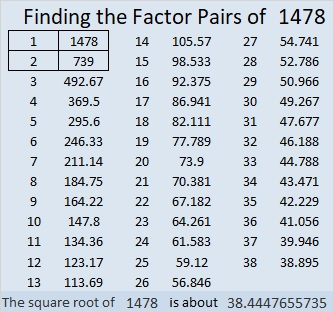### A little more about the number 1478:

1478 is not the sum or the difference of two squares, but it is the short leg in a Pythagorean triple:
(1478-546120-546122) calculated from 2(739)(1), 739² – 1², 739² + 1².

# 349 and Level 6

When 2^349 is divided by 349, the remainder is 2, so 349 is VERY LIKELY a prime number. Scroll down past the puzzle to know for sure.Print the puzzles or type the factors on this excel file: 10 Factors 2015-01-05

• 349 is a prime number.
• Prime factorization: 349 is prime.
• The exponent of prime number 349 is 1. Adding 1 to that exponent we get (1 + 1) = 2. Therefore 349 has exactly 2 factors.
• Factors of 349: 1, 349
• Factor pairs: 349 = 1 x 349
• 349 has no square factors that allow its square root to be simplified. √349 ≈ 18.682

How do we know that 349 is a prime number? If 349 were not a prime number, then it would be divisible by at least one prime number less than or equal to √349 ≈ 18.682. Since 349 cannot be divided evenly by 2, 3, 5, 7, 11, 13, or 17, we know that 349 is a prime number.Prime Number Trivia: There are sixteen prime numbers between 300 and 400. Of those, 349 is the only one that doesn’t produce another prime number when 300 is subtracted from it. 349 – 300 = 49, a composite number. This piece of trivia might be helpful if you wanted to memorize all the prime numbers less than 1000.# 348 and Level 5

Quick Prime/Composite Number Test: 348 is even and thus divisible by 2, so 348 is a composite number.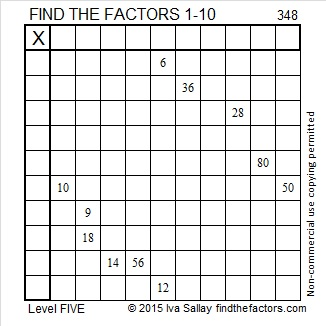Print the puzzles or type the factors on this excel file: 10 Factors 2015-01-05

• 348 is a composite number.
• Prime factorization: 348 = 2 x 2 x 3 x 29, which can be written 348 = (2^2) x 3 x 29
• The exponents in the prime factorization are 2, 1, and 1. Adding one to each and multiplying we get (2 + 1)(1 + 1)(1 + 1) = 3 x 2 x 2 = 12. Therefore 348 has exactly 12 factors.
• Factors of 348: 1, 2, 3, 4, 6, 12, 29, 58, 87, 116, 174, 348
• Factor pairs: 348 = 1 x 348, 2 x 174, 3 x 116, 4 x 87, 6 x 58, or 12 x 29
• Taking the factor pair with the largest square number factor, we get √348 = (√4)(√87) = 2√87 ≈ 18.655# Prime Number Tests for 347

• 347 is a prime number.
• Prime factorization: 347 is prime.
• The exponent of prime number 347 is 1. Adding 1 to that exponent we get (1 + 1) = 2. Therefore 347 has exactly 2 factors.
• Factors of 347: 1, 347
• Factor pairs: 347 = 1 x 347
• 347 has no square factors that allow its square root to be simplified. √347 ≈ 18.628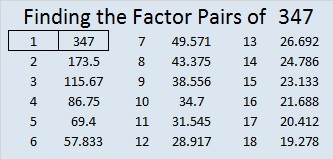How do we know that 347 is a prime number? If 347 were not a prime number, then it would be divisible by at least one prime number less than or equal to √347 ≈ 18.628. Since 347 cannot be divided evenly by 2, 3, 5, 7, 11, 13, or 17, we know that 347 is a prime number.

Is there a quicker way to tell that 347 is a prime number? Not exactly…There is a quick test that will tell you if 347 is VERY LIKELY prime.

Quick Prime or Composite Number Test: You can use as few as TEN calculator keystrokes to test if 347 is VERY LIKELY prime: What is the remainder when 2³⁴⁷ is divided by 347? To find out type “(2)(xʸ)(3)(4)(7)(Mod)(3)(4)(7)” followed by the equal sign into your computer’s calculator. This is how the calculator should look before you hit the equal sign:2^347 mod (347) = 2, the same as the base we typed in. That means 347 is VERY LIKELY prime. This tests works for all prime numbers, but sometimes it gives a false positive for a relatively few (but infinite number) of composite numbers. (341 is the smallest of these numbers, and I wrote about it here.)

2 isn’t the only prime number that can be used as the base in this quick test. You can verify the following also on your computer’s calculator:

• 3^347 mod (347) = 3
• 5^347 mod (347) = 5
• 7^347 mod (347) = 7
• 11^347 mod (347) = 11. We can practically go on forever using prime number after prime number….until
• 337^347 mod (347) = 337, the largest prime number less than 347.

As long as we use a prime less than 347 as the base and 347 as the exponent, we will always get that prime as the remainder. Still even though 347 passes ALL those prime number tests, we can only conclude that 347 is VERY LIKELY prime. Doing all those calculations is more work than simply dividing 347 by all the prime numbers less than or equal to its square root and getting a remainder every single time.

Tomcircle, a fellow blogger, shared a video of a new-prime-number-test that works for prime numbers and NEVER gives a false positive.

While this test works every time in theory, it can be quite a nightmare in practice. It involves putting the possible prime number into a particular expression, expanding the expression, calculating each coefficient, and verifying that all those coefficients are divisible by this possible prime number. In the case of the relatively small number 347, there would be 173 different coefficients. Each of those coefficients are the numbers in the 347th row of Pascal’s triangle, and too many of them are usually expressed in scientific notation. Dividing each of them by 347 to verify there is no remainder is far more work than most people would want to do. In fact, dividing 347 by all the integers less than 347 would actually be less work!

The Sieve of Eratosthenes was how the ancient Greeks found prime numbers. It takes a little longer than dividing the possible prime number by all of the primes less than or equal to its square root, but it finds many primes at the same time. Solvemymath shares some interesting facts about it.

Prime numbers are intriguing, and 347 is one of them!

# 346 and Level 4

Quick Prime/Composite Number Test: 346 is even and thus divisible by 2, so 346 is a composite number.Print the puzzles or type the factors on this excel file: 10 Factors 2015-01-05

• 346 is a composite number.
• Prime factorization: 346 = 2 x 173
• The exponents in the prime factorization are 1 and 1. Adding one to each and multiplying we get (1 + 1)(1 + 1) = 2 x 2 = 4. Therefore 346 has exactly 4 factors.
• Factors of 346: 1, 2, 173, 346
• Factor pairs: 346 = 1 x 346 or 2 x 173
• 346 has no square factors that allow its square root to be simplified. √346 ≈ 18.601# 345 and Level 3

Quick Prime or Composite Number Test: The last digit of 345 is 5, so it can be evenly divided by 5. Therefore, 345 is a composite number.Print the puzzles or type the factors on this excel file: 10 Factors 2015-01-05

• 345 is a composite number.
• Prime factorization: 345 = 3 x 5 x 23
• The exponents in the prime factorization are 1, 1, and 1. Adding one to each and multiplying we get (1 + 1)(1 + 1)(1 + 1) = 2 x 2 x 2 = 8. Therefore 345 has exactly 8 factors.
• Factors of 345: 1, 3, 5, 15, 23, 69, 115, 345
• Factor pairs: 345 = 1 x 345, 3 x 115, 5 x 69, or 15 x 23
• 345 has no square factors that allow its square root to be simplified. √345 ≈ 18.574A Logical Approach to FIND THE FACTORS: Find the column or row with two clues and find their common factor. Write the corresponding factors in the factor column (1st column) and factor row (top row).  Because this is a level three puzzle, you have now written a factor at the top of the factor column. Continue to work from the top of the factor column to the bottom, finding factors and filling in the factor column and the factor row one cell at a time as you go.# 344 and Level 2

Quick Prime or Composite Number Test: 344 is even and thus divisible by 2, so 344 is a composite number.Print the puzzles or type the factors on this excel file: 10 Factors 2015-01-05

• 344 is a composite number.
• Prime factorization: 344 = 2 x 2 x 2 x 43, which can be written (2^3) x 43
• The exponents in the prime factorization are 3 and 1. Adding one to each and multiplying we get (3 + 1)(1 + 1) = 4 x 2 = 8. Therefore 344 has exactly 8 factors.
• Factors of 344: 1, 2, 4, 8, 43, 86, 172, 344
• Factor pairs: 344 = 1 x 344, 2 x 172, 4 x 86, or 8 x 43
• Taking the factor pair with the largest square number factor, we get √344 = (√4)(√86) = 2√86 ≈ 18.547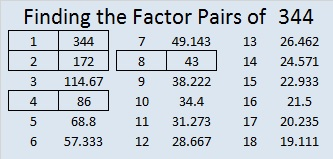# 343 and a Few Changes

Starting today, I’ve made a few small changes to the way my posts will look. If you can spot what the changes are, let me know if you have an opinion about them.

Quick Prime or Composite Number Test: If you divide 2^343 by 343, the remainder is 324, not 2, so 343 is NOT a prime number and is a composite number. (Use the mod function on the computer’s calculator to find the remainder.) Scroll down to see 343’s factors.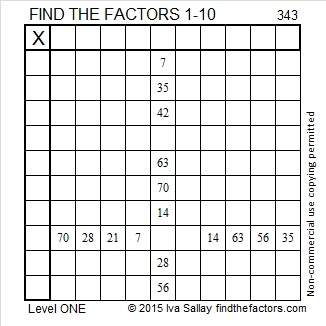Print the puzzles or type the factors on this excel file: 10 Factors 2015-01-05

• 343 is a composite number.
• Prime factorization: 343 = 7 x 7 x 7, which can be written 343 = 7³
• The exponent in the prime factorization is 3. Adding one, we get (3 + 1) = 4. Therefore 343 has exactly 4 factors.
• Factors of 343: 1, 7, 49, 343
• Factor pairs: 343 = 1 x 343 or 7 x 49
• Taking the factor pair with the largest square number factor, we get √343 = (√7)(√49) = 7√7 ≈ 18.520
• 343 is a perfect cube.OEIS.org tells us that (3+4)³ = 343, so 343 is a Friedman number, a number that can be expressed using only its own digits and (), +, -, ×, and ÷.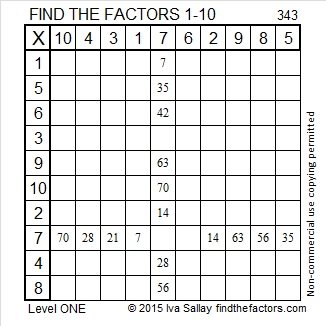# 342 and Level 6

• 342 is a composite number.
• Prime factorization: 342 = 2 x 3 x 3 x 19, which can be written 342 = 2 x (3^2) x 19
• The exponents in the prime factorization are 1, 2, and 1. Adding one to each and multiplying we get (1 + 1)(2 + 1)(1 + 1) = 2 x 3 x 2 = 12. Therefore 342 has exactly 12 factors.
• Factors of 342: 1, 2, 3, 6, 9, 18, 19, 38, 57, 114, 171, 342
• Factor pairs: 342 = 1 x 342, 2 x 171, 3 x 114, 6 x 57, 9 x 38, or 18 x 19
• Taking the factor pair with the largest square number factor, we get √342 = (√9)(√38) = 3√38 ≈ 18.493342 = 18 × 19, which means it is the sum of the first 18 even numbers.

• Thus, 2 + 4 + 6 + 8 + . . .  + 32 + 34 + 36 = 342

It also means that we are halfway between 18² and 19², or halfway between 324 and 361. The average of those two numbers is 342.5.

AND it means that 18² + 19² – 1 = 2(342) = 2(18 × 19)

Here are a few things to think about before you try to solve the puzzle I’ve posted below:

• Will you decide to use the 6 or the 9 as the common factor of 72 and 54?
• Will 5 or 10 be the common factor of 20 and 50?
• The common factor for 27 and 9 could be 9 or it could be 3,
• and the common factor for 32 and 24 is either 4 or 8.
• Can you use logic to come up with the correct factor choices to make this puzzle work as a multiplication table?Print the puzzles or type the factors on this excel file: 12 Factors 2014-12-29# 341 is the smallest composite number that gives a false positive for this Quick Prime Number Test

• 341 is a composite number.
• Prime factorization: 341 = 11 x 31
• The exponents in the prime factorization are 1 and 1. Adding one to each and multiplying we get (1 + 1)(1 + 1) = 2 x 2 = 4. Therefore 341 has exactly 4 factors.
• Factors of 341: 1, 11, 31, 341
• Factor pairs: 341 = 1 x 341 or 11 x 31
• 341 has no square factors that allow its square root to be simplified. √341 ≈ 18.466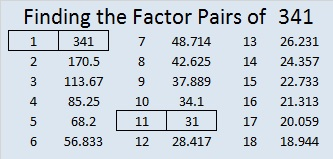341 is a composite number that sometimes acts like a prime number. To understand why, we need to understand a little bit about modular arithmetic:

When one number is divided by another, sometimes there is a remainder. Modular arithmetic is all about the remainders. We don’t care how many times one number divides into another number; we only care about the remainder.

Something very curious happens when the equation in the chart below is applied to a prime number greater than 2: The remainder is always 2. It really is! For example, 2^5 = 32 and 32 divided by 5 is 6R2. We say that 32 (mod 5) = 2 or 2^5 (mod 5) = 2 because 2 is the remainder. The fact that the remainder for prime numbers applied to this equation is always 2 is amazing, and can be a QUICK TEST to see if an odd number might be a PRIME NUMBER! If the remainder isn’t 2, that odd number is definitely NOT prime!

Passing the remainder test is a necessary but not a sufficient indicator that a number is prime: Even though 341 is not a prime number, the quantity 2^341 divided by 341 also has a remainder of 2. Since 341 = 11 x 31, but passes the remainder test, it is known as a pseudoprime number.  341 is the smallest composite number that passes this particular test, so 341 is an amazing number!

Also in the chart above, 2 is the most common remainder, followed by 8, then 32, then 128. All of those numbers are odd powers of 2. The even powers of 2 do not appear on the chart at all! That is a very curious phenomenon as well. (However, if x is an even number, it appears that y will usually be an even power of 2.)

Earlier mathematicians have written equivalent expressions and algorithms, but I prefer using “2^x (mod x)” because it takes very few keystrokes to enter into a calculator before hitting the equal sign:

Look at the image below.  It demonstrates that in prime-numbered rows, the numbers in that row can be divided evenly by that prime number (not counting the 1’s at the beginning and ending of each row).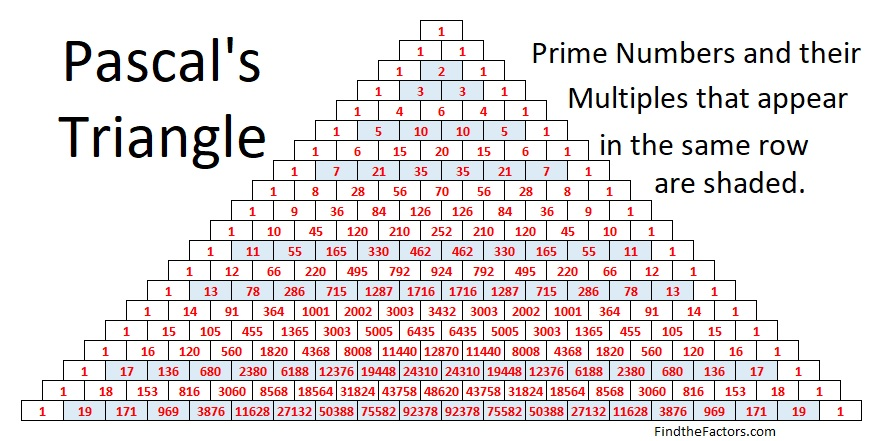• For a composite number, such as 15, at least one of the numbers in the row will NOT be divisible by that composite number. In row 15, 1 is the 0th term, 15 is the 1st term, 105 is the 2nd term, 455 is the 3rd term, and 1365 is the 4th term and so forth.
• Terms that are divisible by 15 are the 1st term (15), the 2nd term (105), the 4th term (1365), the 7th term (6435), the 8th term (6435), the 11th term (1365), and the 13th term (105). All of those term numbers, 1, 2, 4, 7, 8, 11, and 13, do NOT have factors in common with the number 15.
• The terms that are NOT divisible by 15 are the 3rd term (455), the 5th term (3003), the 6th term (5005), the 9th term (5005), the 10th term (3003), and the 12th term (455). All of those term numbers, 3, 5, 6, 9, 10, and 12, have at least one factor in common with the number 15.

If we could see the VERY large numbers for the 341st row, and if they weren’t expressed in Scientific Notation, we could note that the following terms would NOT be divisible by 341: terms numbered 11th, 22nd, 33rd, 44th, and so forth and the terms numbered 31st, 62nd, 93rd and so forth. However, if you add those terms together, that very large sum would be divisible by 341. That is so amazing, even though 341 is not prime!

This second image from Pascal’s Triangle demonstrates that the sum of the numbers in any row of Pascal’s triangle equals two raised to the second number in that row.
(There is no second number in the top row, so we could say that second number is zero, and 2º = 1.)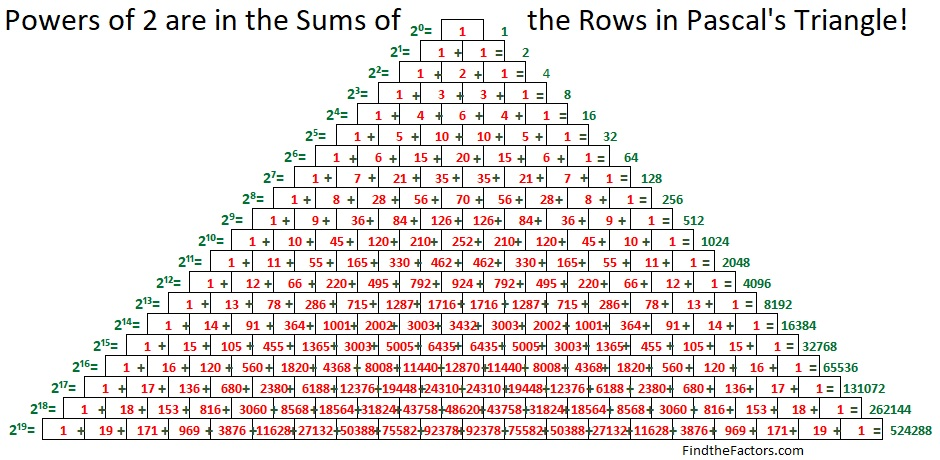These two images work together so that 2^p (mod p) will always be 2 for every prime number greater than 2 because every number in the prime-numbered rows can be evenly divided by that prime number. (Except the 1 at the beginning of the row and the 1 at the end of the row; Note 1 + 1 = 2)

Now that is why this amazing test for prime numbers works as well as it does while giving just a few false positives!